Durer and conic sections (II): ellipses

Albrecht Dürer (1471-1528) was a German painter and engraver. In 1525 Dürer published the first mathematical book in German, the "Four Books on Measurement" or "Course in the Art of Measurement with Compass and Ruler". (Underweysung der Messung mit dem Zirckel und Richtscheyt).

One of the most interesting features of the First Book is the first discussion in German of conic sections.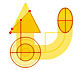Durer was the first who published in german a method to draw ellipses as cone sections.

His beautiful and original method uses a side view and a view from above of a cone cut by an oblique plane.DISCUSSION

Some publications accuse Dürer of having made big mistakes drawing ellipses. Here we can read two opinions defending Dürer's work. These points of view are more balance and well documented.

Dan Pedoe wrote: "The ellipse shown is egg-shaped, whereas a true ellipse has two axes of symmetry. It has been thought that Dürer, like any schoolboy, felt that an ellipse was bound to widen with the widening of the cone of which it is a section, and Kepler, a hundred years later, was amused by this apparent error, although much impressed with Dürer's work. Dürer, wishing to find a German word for ellipsis, did coin the word Eierlinie, egg-line, but on the other hand if one repeats the construction, a minute error will produce the egg-shape effect, and I am not convinced that Dürer was misled. It was Kepler, by the way, who first determined that the orbits of the planets are ellipses, and not epicycles, and can therefore be said to have established the ellipse as a fundamental curve." (Dan Pedoe, Geometry and the Visual Arts)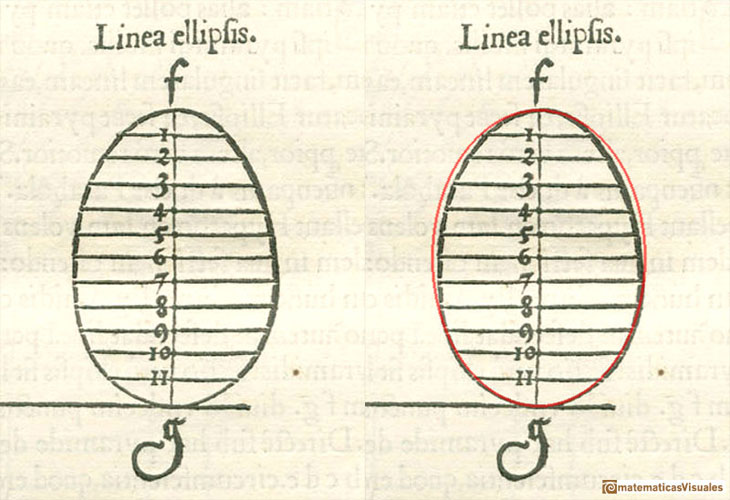Dirk Huylebrouck makes a strong defense of Dürer in front of the accusations that he would have drawn erroneous curves. "The sole valid arguments of the critics, is Dürer's drawing on page 30 of his book 'Underweysung der Messung' or 'Teaching Measurements' (Nürnberg, 1525). It shows a drawing for the plane intersection of a cone. It would become a classic in all courses on Monge's projection method. In this drawing the ellipse is indeed slightly wider where the cone is wider. The critics state that Dürer made 'an elaborate technique to accurately draw the ellipse', but anyone who followed descriptive geometry in high school would laugh away the adjetive 'elaborate'. The logic of the method is simple, but the execution is more problematic. In fact, anyone who ever made this drawing with compass, ruler and pencil experienced it is not so easy get a smooth ellipse. One easily gets and egg shape or else an ellipse with sharp vertices on the longer axis. (...) Knowing the techniques and materials at his disposal, his drawings surely was good enough for any teacher of Monge geometry. It looks slightly like an egg, sure, but it is not exaggeratedly wrong. (...) Perhaps we are too spoiled today by computer drawings to undertand the technical difficulties when making such a drawing by hand".

He wrote that "there is no proof at all Dürer thought an elliptic intersection gets broader where the cone gets broader. He never said so".

Now we are going to prove that the minor axis of an ellipse is a symmetry axis.

INVESTIGATE

Try to prove this result before continuing reading. We need only basic Mathematics to do that.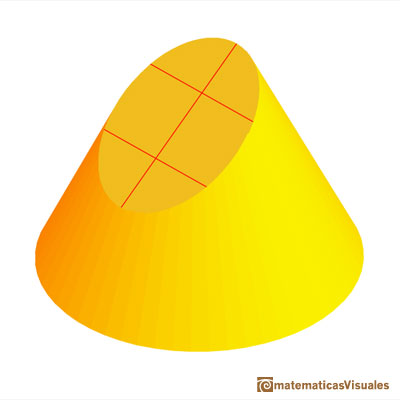First, for each point on the major axis we need to calculate k, how wide is the ellipse at this point.It could be useful to review the geometric mean of two numbers. I used this idea when we studied the sphere.We want to calculate the surface area of sections of a sphere using the Pythagorean Theorem. We also study the relation with the Geometric Mean and the Right Triangle Altitude Theorem.

Now, we consider two symmetric points on the major axis of the ellipse.We must prove that

Or, simply

In the next image there are highlighted two similar triangles.Then

Here we can see two more similar triangles.And we can write

Mixing these two equalities

And then

This is what we wanted to prove.

REFERENCES

Erwin Panofsky, The Life and Art of Albrecht Dürer.
Dan Pedoe, Geometry and the Visual Arts. St Martin's Press Inc, New York, 1976.
Dirk Huylebrouck, 500 Years of Melancholia.
C. Stanley Ogilvy, Excursions in Geometry. Oxford University Press.
Tom Apostol, Calculus. John Wiley and Sons, 1967.
Ex Libris: Dandelin Spheres and the Conic Sections. With a link to the original work by Germinal Pierre Dandelin.We want to calculate the surface area of sections of a sphere using the Pythagorean Theorem. We also study the relation with the Geometric Mean and the Right Triangle Altitude Theorem.Every ellipse has two foci and if we add the distance between a point on the ellipse and these two foci we get a constant.Transforming a circle we can get an ellipse (as Archimedes did to calculate its area). From the equation of a circle we can deduce the equation of an ellipse.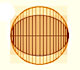In his book 'On Conoids and Spheroids', Archimedes calculated the area of an ellipse. We can see an intuitive approach to Archimedes' ideas.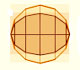In his book 'On Conoids and Spheroids', Archimedes calculated the area of an ellipse. It si a good example of a rigorous proof using a double reductio ad absurdum.An Ellipsograph is a mechanical device used for drawing ellipses.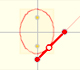If a straight-line segment is moved in such a way that its extremities travel on two mutually perpendicular straight lines then the midpoint traces out a circle; every other point of the line traces out an ellipse.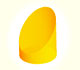We study different cylinders cut by an oblique plane. The section that we get is an ellipse.The section of a cylinder by a plane cutting its axis at a single point is an ellipse. A beautiful demonstration uses Dandelin Spheres.He studied transformations of images, for example, faces.In his book 'Underweysung der Messung' Durer draw a non-regular pentagon with ruler and a fixed compass. It is a simple construction and a very good approximation of a regular pentagon.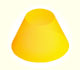Plane developments of cones and conical frustum. How to calculate the lateral surface area.We study different cylinders and we can see how they develop into a plane. Then we explain how to calculate the lateral surface area.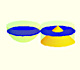Using Cavalieri's Principle we can calculate the volume of a sphere.